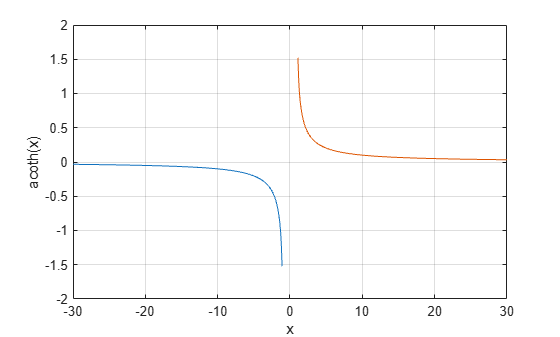# acoth

## 语法

``Y = acoth(X)``

## 说明

``Y = acoth(X)` 返回 `X` 各元素的反双曲余切。该函数同时接受实数和复数输入。所有的角度都以弧度表示。`

## 示例

```X = [2 -3 1+2i]; Y = acoth(X)```
```Y = 1×3 complex 0.5493 + 0.0000i -0.3466 + 0.0000i 0.1733 - 0.3927i ```

$-30\le x<-1$$1 区间上绘制反双曲余切函数。

```x1 = -30:0.1:-1.1; x2 = 1.1:0.1:30; plot(x1,acoth(x1),x2,acoth(x2)) grid on xlabel('x') ylabel('acoth(x)')```## 详细信息

### 反双曲余切

`${\mathrm{coth}}^{-1}\left(x\right)={\mathrm{tanh}}^{-1}\left(\frac{1}{x}\right)=\frac{1}{2}\mathrm{log}\left(\frac{x+1}{x-1}\right).$`